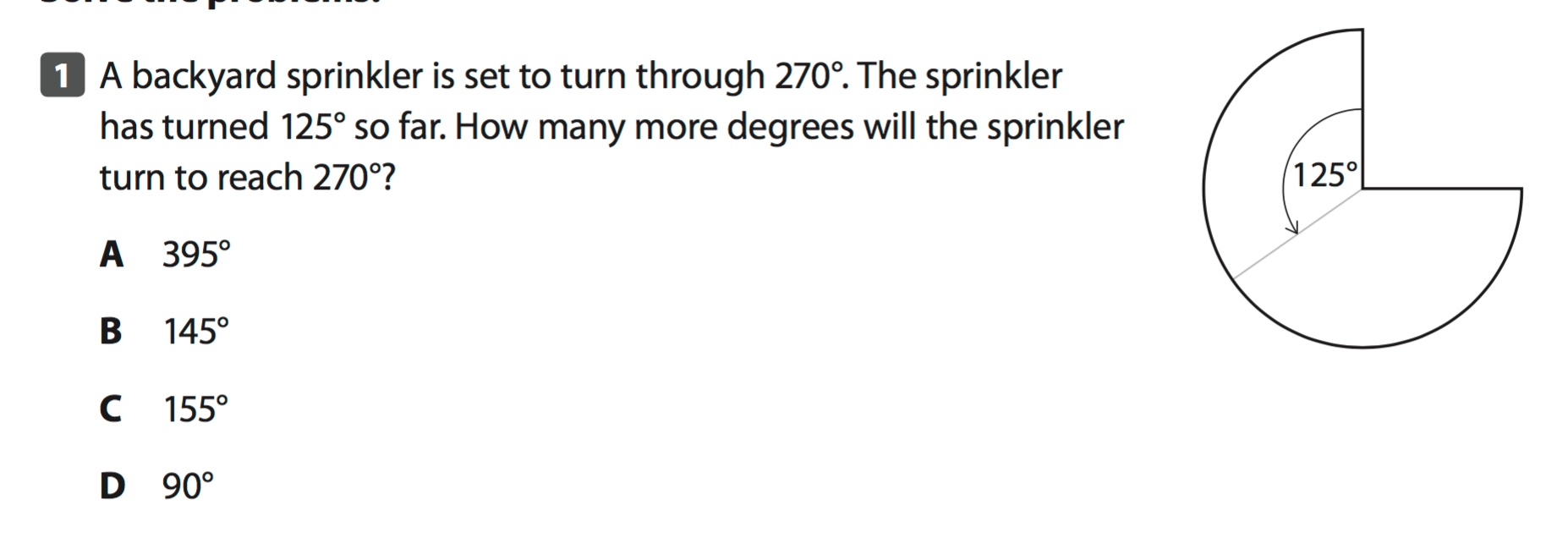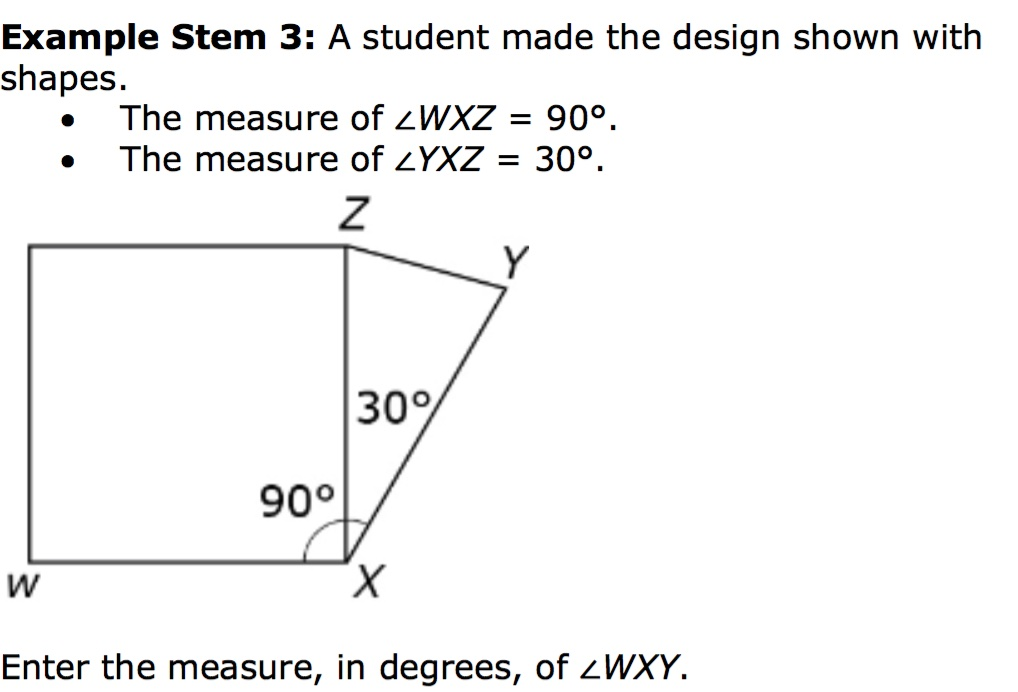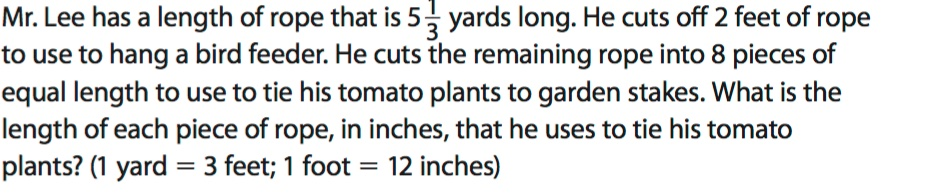Measurement and Data Test
starstarstarstarstarstarstarstarstarstar
by Bani Maita-Perez [Bailey MS]
| 14 Questions1
1
1
How many more degrees will the sprinkler turn to reach 270 degrees.
A 395 degrees
B 145 degrees
C 155 degrees
D 90 degrees2
2
1
Complete the table below to show the amount of each color of paint the worker had in gallons, quarts, and ounces and how many 8 ounce jars of each color paint the worker filled.

Key: ( 1 gallon = 4 quarts)
( 1 quart = 32 fluid ounces)3
3
1
Part A
Place an "X" above the correct tick marks to complete the line plot that displays all of the data.
Use the line plot you made in Part A to answer question 4, 5, and 6.
4
1
Part B
Use the line plot you made in Part A.
Which weight has the greatest amount of books?
5
1
Part C
Use the line plot you made in Part A.
What is the difference in weight between the heaviest and lightest books?
6
1
Part D
Use the line plot you made in Part A.
What is the total weight of all the books?
a. 7 3/8 pounds
b. 8 2/8
c. 3 30/8
d. 6 4/8
7
1
The community center has a back deck with a length of 16 feet and a width of 14 feet. Workers are going to install a side deck that is half the length and half the width of the back deck. What will be the perimeter and area of the side deck?
a. Area = 704 feet squared and Perimeter = 60 feet
b. Area = 56 feet squared and Perimeter = 30 feet
c. Area = 60 feet squared and Perimeter = 30 feet
d. Area = 190 feet squared and Perimeter = 60 feet
8
1
What is the measure of the angle below? Use a protractor to measure.9
9
1
How long is the play without the breaks for intermission?
Choose the letters for all that apply.
A 70 minutes
B 110 minutes
C 230 minutes
D 1 hour, 10 minutes
E 1 hour, 50 minutes10
10
1
Choose the correct measure for <WXY.
A 100 degrees
B 110 degrees
C 90 degrees
D 120 degrees.
11
1
Use the line tool to draw a 145 degree angle.12
12
1
What is the length of each piece of rope, in inches, that he uses to tie his tomato plants?

Key: ( 1 yard = 3 feet)
( 1 foot = 12 inches)13
13
1
Use the model to Choose True or False for each letter.
14
1
A pound of grapes costs \$2.49. Lucas bought two pounds of grapes. He gave the clerk a \$10.00 bill. How much change did Lucas get?
a. \$ 7.51
b. \$ 5.02
c. \$12.49
d. \$20.28